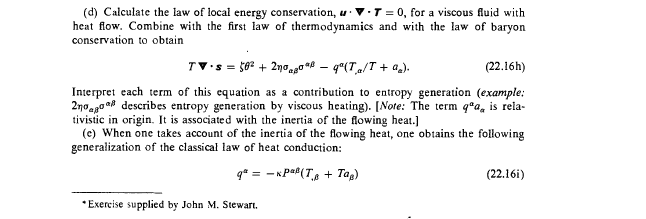# MTW Exercise 22.7 -- Calculate the law of local energy conservation for a viscous fluid with heat flow

Gold Member
Homework Statement:
MTW Ex 22.7(d)
Relevant Equations:
(22.16h) - See attachment
I've come to a grinding halt with this and I can't see a way forward.

Can someone please take a look at what I've done so far and let me know if what I have done is OK and then if it is, give me a hint on how to proceed.

First up,

Is ## u \cdot \nabla \cdot T = u_\alpha T^{\alpha\beta}{}_{;\beta} ## ?

If it is, then from (22.16d)

##u_\alpha T^{\alpha\beta}{}_{;\beta} = u_{\alpha}(\rho u^\alpha u^\beta + (p - \zeta \theta)P^{\alpha \beta} -2\eta \sigma ^{\alpha \beta} +q^{\alpha} u^{\beta} +u^{\alpha} q^{\beta})_{;\beta}##

Working through the derivative and the projections, I reach

##u_\alpha T^{\alpha\beta}{}_{;\beta} = \rho \theta + u^{\beta}\rho_{;\beta} + u^i((p - \zeta \theta)_{;i} + \eta u^i(\frac{1}{3} \theta_{;i}) + u_i u^{\beta} q^i{}_{;\beta} = u_i q^i \theta -q^i{}_{;i}##

Where ##\theta = u^{\alpha}{}_{;\alpha}##

Is that OK?

Regards

TerryWLast edited by a moderator:

etotheipi
Hey, sorry you haven't been getting any replies. I must admit I don't have much of a clue either. Here's just some things I was thinking, but I can't guarantee any of its correctness. $$T \nabla \cdot \mathbf{s} = T \partial_{\mu} s^{\mu} = T\partial_{\mu} (nsu^{\mu} + \frac{1}{T} q^{\mu})$$By baron number conservation you have ##\nabla \cdot \mathbf{S} = \partial_{\mu}(n u^{\mu}) = 0##, thus you can re-write this as$$T\nabla \cdot \mathbf{s} = nT u^{\mu} \partial_{\mu} s + \partial_{\mu} q^{\mu} - \frac{1}{T} q^{\mu} \partial_{\mu} T \ \ \ (1)$$The first law of thermodynamics is$$u^{\mu} \partial_{\mu} \rho = \frac{\rho + p}{n} u^{\mu} \partial_{\mu} n + nT u^{\mu} \partial_{\mu} s$$Inserting this into ##(1)## and re-writing ##u^{\mu}\partial_{\mu} n = -n \partial_{\mu} u^{\mu}##gives$$T\nabla \cdot \mathbf{s} = u^{\mu} \partial_{\mu} \rho + (\rho + p) \partial_{\mu} u^{\mu} + \partial_{\mu} q^{\mu} - \frac{1}{T} q^{\mu} \partial_{\mu} T$$Now look at the local energy conservation equation ##\mathbf{u} \cdot \nabla \cdot \boldsymbol{\mathcal{T}} = 0## with\begin{align*} \boldsymbol{\mathcal{T}} &= - 2 \eta \boldsymbol{\sigma} - \boldsymbol{\zeta} \theta \mathbf{P} + \mathbf{u} \otimes \mathbf{q} + \mathbf{q} \otimes \mathbf{u} + (p+\rho) \mathbf{u} \otimes \mathbf{u} + \rho \mathbf{g} \\ \\ \mathcal{T}^{\mu \nu} &= -2 \eta \sigma^{\mu \nu} - \zeta \theta P^{\mu \nu} + u^{\mu} q^{\nu} + q^{\mu} u^{\nu} + (p + \rho) u^{\mu} u^{\nu} + \rho g^{\mu \nu} \end{align*}Then you have\begin{align*} \mathbf{u} \cdot \nabla \cdot \boldsymbol{\mathcal{T}} = -2\eta u_{\nu} \partial_{\mu} \sigma^{\mu \nu} - \zeta u_{\nu} \partial_{\mu} (\theta P^{\mu \nu}) + u_{\nu} \partial_{\mu}(u^{\mu} q^{\nu}) + u_{\nu} \partial_{\mu}(q^{\mu} u^{\nu}) \\ + \, u_{\nu} \partial_{\mu}((p + \rho) u^{\mu} u^{\nu}) + u_{\nu} \partial_{\mu}(\rho g^{\mu \nu}) \overset{!}{=} 0 \end{align*}where in a local Lorentz frame you have ##\theta = \partial_{\alpha} u^{\alpha}## and also$$\sigma^{\mu \nu} = \frac{1}{2}(P^{\alpha \nu} \partial_{\alpha} u^{\mu} + P^{\alpha \mu} \partial_{\alpha} u^{\nu}) - \frac{1}{3} P^{\mu \nu} \partial_{\alpha} u^{\alpha}$$I'm not sure how to continue. There's some bits and pieces you can tidy up, using e.g. the metric compatibility ##\partial_{\mu} g^{\mu \nu} = 0## and other identities, but I don't know. Can someone else advise?

Gold Member
Hi Etotheipi,

Thanks for getting back to me on this.

I'll spend a bit of time comparing your working with mine to see if it generates any further ideas

Inserting this into (1) and re-writing uμ∂μn=−n∂μuμgives
I hadn't thought of doing this, I just took ##\partial_{\mu} (nu^{\mu}) = 0 ##

where in a local Lorentz frame you have θ=∂αuα and also

I know that the Lorentz frame was used earlier to help arrive at a solution but I wonder if it can help here as reducing ##u \cdot \nabla \cdot T## to the Lorentz frame produces a quite simple equation which doesn't look like it will help me get anywhere.

I tried working back starting with ##\sigma_{\alpha \beta} \sigma ^{\alpha \beta}## but that didn't produce anything that looked useful.

I had a chat with my son last night. He read Astrophysics at King's and knew John Stewart. His main comment was the John Stewart was infamous for setting impossible exam questions!

I'll post again if I make any further progress.

Regards

TerryW

•etotheipi